Get Free NCERT Solutions for Class 10 Maths Chapter 6 Ex 6.2 PDF. Triangles Class 10 Maths NCERT Solutions are extremely helpful while doing your homework. Exercise 6.2 Class 10 Maths NCERT Solutions were prepared by Experienced LearnCBSE.in Teachers. Detailed answers of all the questions in Chapter 6 Maths Class 10 Triangles Exercise 6.2 provided in NCERT TextBook.

Free download NCERT Solutions for Class 10 Maths Chapter 6 Exercise 6.2 Triangles PDF in Hindi Medium as well as in English Medium for CBSE, Uttarakhand, Bihar, MP Board, Gujarat Board, AP SSC, TS SSC and UP Board students, who are using NCERT Books based on updated CBSE Syllabus for the session 2019-20.

You can also download the free PDF of Ex 6.2 Class 10 Triangles NCERT Solutions or save the solution images and take the print out to keep it handy for your exam preparation.

 Board CBSE Textbook NCERT Class Class 10 Subject Maths Chapter Chapter 6 Chapter Name Triangles Exercise Ex 6.2 Number of Questions Solved 10 Category NCERT Solutions

## NCERT Solutions For Class 10 Maths Chapter 6 Triangles Ex 6.2

NCERT Solutions for Class 10 Maths Chapter 6 Triangles Ex Ex 6.2 are part of NCERT Solutions for Class 10 Maths. Here we have given NCERT Solutions for Class 10 Maths Chapter 6 Triangles Exercise 6.2

Question 1.
In the given figure (i) and (ii), DE || BC. Find EC in (i) and AD in (ii).Solution: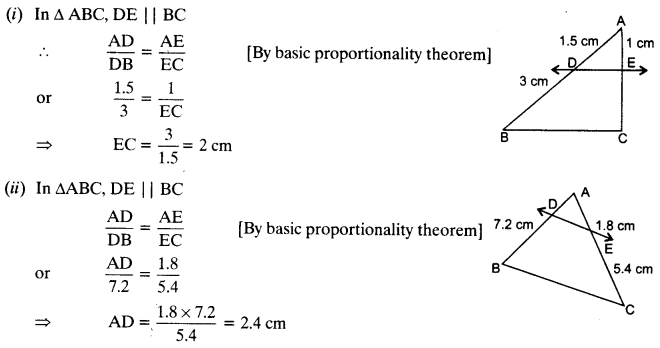Question 2.
E and F are points on the sides PQ and PR respectively of a ∆PQR. For each of the following cases, state whether EF || QR:
(i) PE = 3.9 cm, EQ = 3 cm, PF = 3.6 cm and FR = 2.4 cm
(ii) PE = 4 cm, QE = 4.5 cm, PF = 8 cm and RF = 9 cm
(iii) PQ = 1.28 cm, PR = 2.56 cm, PE = 0.18 cm and PF = 0.36 cm
Solution: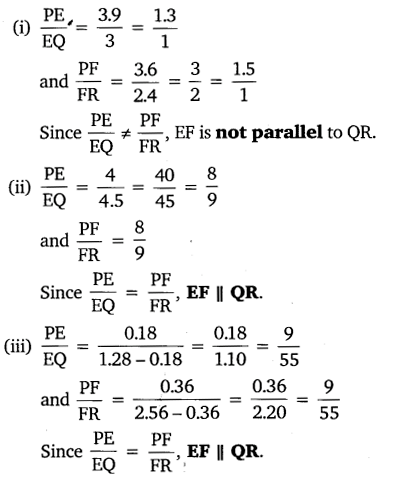Question 3.
In the given figure, if LM || CB and LN || CD.
Prove that $$\frac { AM }{ AB } =\frac { AN }{ A{ D }^{ \bullet } }$$Solution: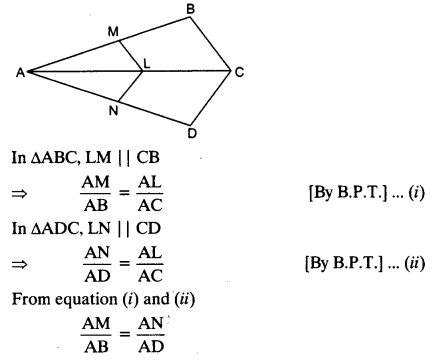Question 4.
In the given figure, DE || AC and DF || AE.
Prove that $$\frac { BF }{ FE } =\frac { BE }{ E{ C }^{ \bullet } }$$Solution: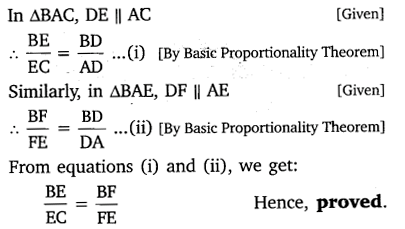Question 5.
In the given figure, DE || OQ and DF || OR. Show that EF || QR.Solution: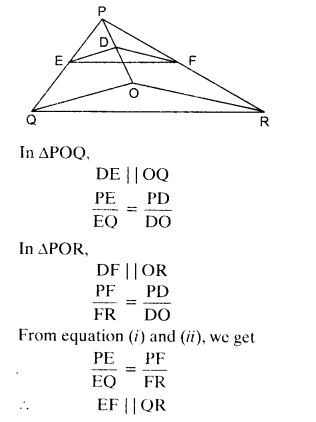Question 6.
In the given figure, A, B and C are points on OP, OQ and OR respectively such that AB || PQ and AC || PR. Show that BC || QR.Solution:Question 7.
Using B.P.T., prove that a line drawn through the mid-point of one side of a triangle parallel to another side bisects the third side. (Recall that your have proved it in class IX)
Solution: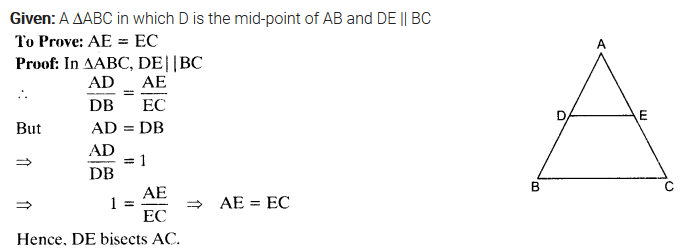Question 8.
Using converse of B.P.T., prove that the line joining the mid-points of any two sides of a triangle is parallel to the third side.  (Recall that your have done it in class IX)
Solution:Question 9.
ABCD is a trapezium in which AB || DC and its diagonals intersect each other at the point O. Show that $$\frac { AO }{ BO } =\frac { CO }{ D{ O }^{ \bullet } }$$
Solution: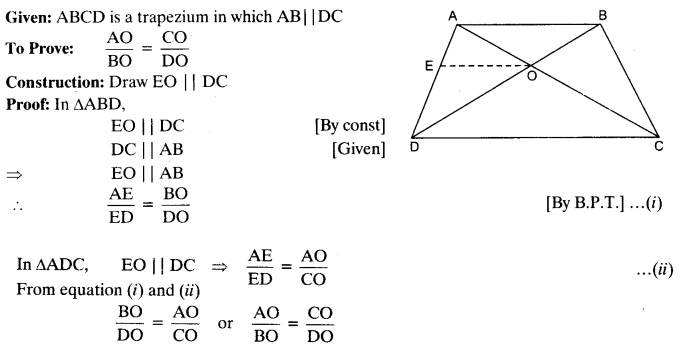Question 10.
The diagonals of a quadrilateral ABCD intersect each other at the point O such that $$\frac { AO }{ BO } =\frac { CO }{ D{ O }^{ \bullet } }$$ Show that ABCD is a trapezium.
Solution: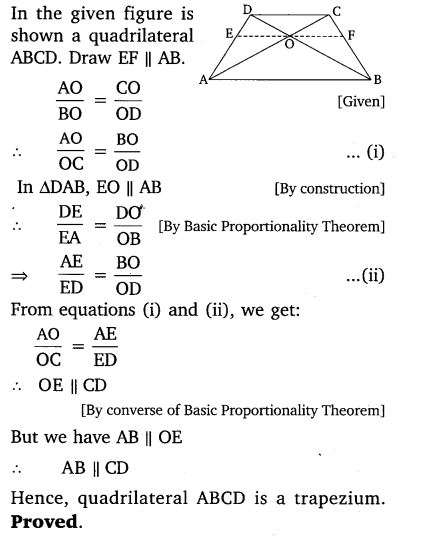## NCERT Solutions for Class 10 Maths Chapter 6 Triangles Ex 6.2 in Hindi Medium

Q1. आकृति 6.17 (i) और (ii) में, DE || BC में AD ज्ञात कीजिए :

हल: (i)

Δ ABC में

DE || BC   दिया है |

अत: आधारभूतिक समानुपातिक प्रमेय सेQ2. किसी त्रिभुज PQR की भुजाओं PQ और PR पर क्रमशः बिन्दु और स्थित हैं |  निम्नलिखित में से प्रत्येक स्थिति के लिएबताइए कि क्या EF|| QR है |

(i)   PE = 3.9 cm, EQ= 3cm, PF = 3.6 और FR= 2.4 cm

(ii)     PE = 4 cm, QE = 4.5 cm, PF = 8 cm और RF = 9 cm

(iii)     PQ = 1.28 cm, PR = 2.56 cm, 0.18 cm और PF = 0.36 cmइसलिए, EF|| QR नहीं है |Q7. प्रमेय 6.1 का प्रयोग करते हुए सिद्ध कीजिए कि एक त्रिभुज की एक भुजा के मध्य -बिन्दु से होकर दूसरी भुजा के समांतर खींची गई रेखा तीसरी भुजा को समद्धिभाजित करती है  | (याद कीजिए की आप इसे कक्षा IX में सिद्ध कर चुके हैं|)​Q8. प्रमेय 6.2 का प्रयोग करते हुए सिद्ध कीजिए की एक त्रिभुज की किन्ही दो भुजाओं के मध्य बिन्दुओं को मिलाने वाली रेखा तीसरी भुजा के समांतर होती है | (याद कीजिए की आप कक्षा IX में ऐसा कर चुके हैं ) |ProvedWe hope the NCERT Solutions for Class 10 Maths Chapter 6 Triangles Ex 6.2, help you. If you have any query regarding NCERT Solutions for Class 10 Maths Chapter 6 Triangles Exercise 6.2, drop a comment below and we will get back to you at the earliest.